Worksheets and No Prep Teaching Resources
Math Worksheets

# Time Worksheets

Few skills are as vital as the ability to tell time, and being on time will help students be successful in whatever career they choose. These worksheets will make sure younger students from preschool through second grade have a firm grasp of this subject. Beautiful graphics combined with meticulous exercises make these worksheets the best way for students to learn all about minutes, hours, and clocks. It's about time!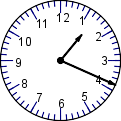Telling Time

Telling Time Math Center (easier - hour hand does not move between numbers)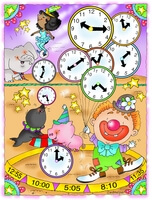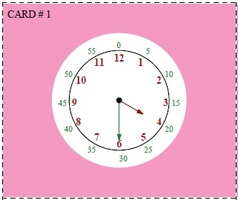Minutes shown on clock (Grades K-1) Normal clock - just hours shown on clock (Grades 1-3)

Telling Time Math Center (more difficult - hour hand moves with minutes like a real clock)Minutes shown on clock (Grades K-1) Normal clock - just hours shown on clock (Grades 1-3)

Telling Time Activity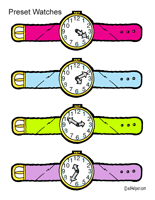Which Watch

Writing Numbers on a Clock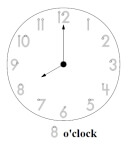Trace all the numbers on a clock

Book Units
Benjamin Banneker: Pioneering Scientist (Grades 2-5)

Missing Number on a Clock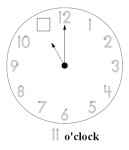Trace numbers on clock and fill in the missing number

Easy Clock and Time Matching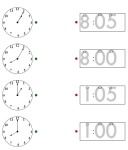Clock Matching: Hours Clock Matching: On the hour and five minutes past given (same hour used)

Clocks for Bulletin Board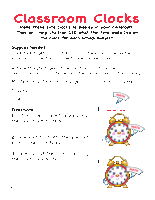Classroom Clocks

Hour Sequence:  Complete the Time Sequence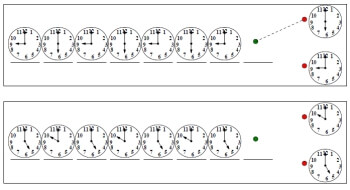Very easy clock sequence - First sequence has a trace answer line Very easy clock sequence - two different hours Very easy clock sequence - three different hours Two or three different hours in sequence More challenging clock hour sequence

Time Logic Printables
Time it took to finish homework
Medical practice: doctors, appointments, and patients
Time watching television
Time watching television (with division)
Bobsled competition

Puzzles Handout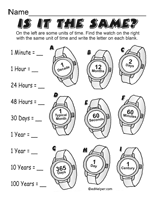Time and Seasons Puzzles

Clock Activity Center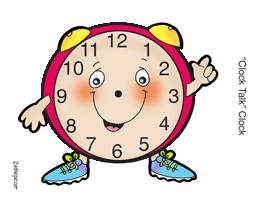Clock Talk

Telling Time (First Grade)
Hour
Write the time (____ o'clock; half past ____)
Write the time (____:____)
Write the time two ways
Show the time on the clock
Write the time two ways (smaller clocks)

Half-Hour
Write the time (half past ____)
Write the time (____:____)
Write the time two ways
Show the time on the clock
Write the time two ways (smaller clocks)

Mixed: Hour and Half-Hour (larger clocks)
Write the time (half past ____ and ____ o'clock)
Write the time (____:____)
Write the time two ways
Show the time on the clock

Mixed: Hour and Half-Hour (smaller clocks)
Write the time (half past ____ and ____ o'clock)
Write the time (____:____)
Write the time two ways
Show the time on the clock
Circle the correct time (2 choices)

Review
Telling Time Mixed Review

Leveled Books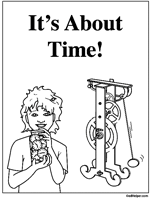It's About Time!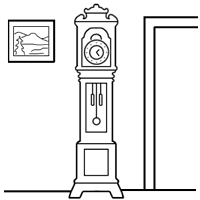My Grandmother's Grandfather Clock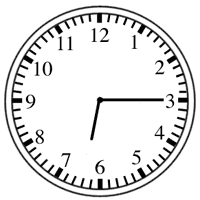What Time Is It?

Telling Time (Second Grade)
Hour and Half-Hour
Write the time (____ o'clock)
Write the time (____:____)
Write the time two ways
Show the time on the clock
Circle the correct time
Write the time (other descriptions used)

Time to 15 Minutes
Write the time (____:____)
Show the time on the clock
Circle the correct time
Write the time (other descriptions used)

Time to 5 Minutes
Write the time (____:____)
Show the time on the clock
Circle the correct time
Write the time (other descriptions used)

Minutes
Write the time (____:____)
Show the time on the clock
Circle the correct time
Write the time (other descriptions used)

How long will it take? (estimating the time needed to do something)
Comparing things to do to one minute
Comparing things to do to 30 minutes
Mixed

Time Word Problems
Time to 10 minutes
Time to 5 minutes
Time to minutes

Time Patterns: Adding 5, 10, 15, 20, 25, and 30 minutes
Digital clocks are all given; need to draw hands on a few clocks
Analog clocks are all given; need to write the time
Complete the pattern

Time Patterns: Subtracting 5, 10, 15, 20, 25, and 30 minutes
Digital clocks are all given; need to draw hands on a few clocks
Analog clocks are all given; need to write the time
Complete the pattern

Time Patterns: Adding and subtracting 5, 10, 15, 20, 25, and 30 minutes
Digital clocks are all given; need to draw hands on a few clocks
Analog clocks are all given; need to write the time
Complete the pattern

Time Patterns (with smaller clocks): Adding and subtracting 5, 10, 15, 20, 25, and 30 minutes
Digital clocks are all given; need to draw hands on a few clocks
Analog clocks are all given; need to write the time
Complete the pattern

Time Patterns: Adding and subtracting 15, 30, 45, 60, 75, 90, 105, and 120 minutes
Digital clocks are all given; need to draw hands on a few clocks
Analog clocks are all given; need to write the time
Complete the pattern

Time Patterns: Adding and subtracting 1, 2, 3, 4, 5, 6, 7, 8, 9, and 10 minutes
Digital clocks are all given; need to draw hands on a few clocks
Analog clocks are all given; need to write the time
Complete the pattern

Elapsed Time
Figure out the elapsed time. Write how much time has passed.

Telling Time Mixed Review
Telling Time Mixed Review

Understanding Time Words
Time Words (Half-Hour and Hour)
Time Words (Five Minutes)
Time Words (Any Time)
Time Words (Five Minutes; May Include "Quarter To")
Time Words (Any Time; May Include "Quarter To")

a.m. and p.m.
Write the Time (ten minutes after seven in the morning is 7:10 a.m.)

Word Problems (with Clock on left)
Clock and Word Problems (Sheet #1)
Clock and Word Problems (Sheet #2)
Clock and Word Problems (Sheet #3)
Clock and Word Problems (Sheet #4)

Elapsed Time
Only Hours
Hours and Half-Hours
15 Minute Intervals
5 Minute Intervals
Any Elapsed Time

Schedules
School Schedule Word Problems

Days of the Week

Time Word Problems from the Math Standardized Test Prep Section
* This section is for edHelper.com subscribers. A subscription to edHelper includes access to the math, spelling, and vocabulary subscription areas. Sign up for the subscriber materials.

Divide the Day Activity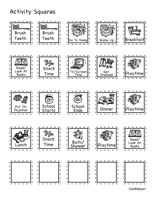A Day Divided

More Activities and Printables
 Calendars
 Clock
 Months
 Times

Have a suggestion or would like to leave feedback?
Leave your suggestions or comments about edHelper!